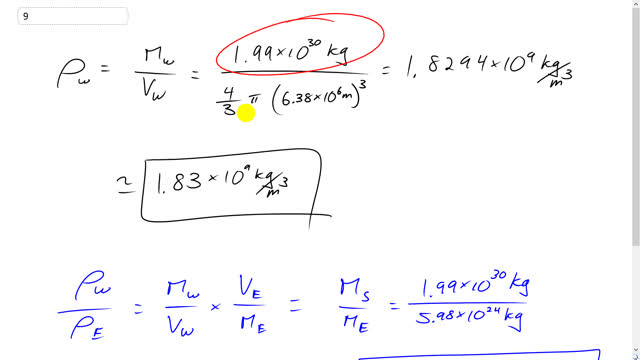## You are here

Question:

Calculate the density of a white dwarf whose mass is equal to the Sun’s and whose radius is equal to the Earth’s. How many times larger than Earth’s density is this?

Giancoli, Douglas C., Physics: Principles with Applications, 7th Ed., ©2014. Reprinted by permission of Pearson Education Inc., New York.
The question will be visible after logging in, as required by Pearson Education Inc.

$\rho_w = 1.83 \times 10^9 \textrm{ kg/m}^3$, $3.3 \times 10^5$ times as large